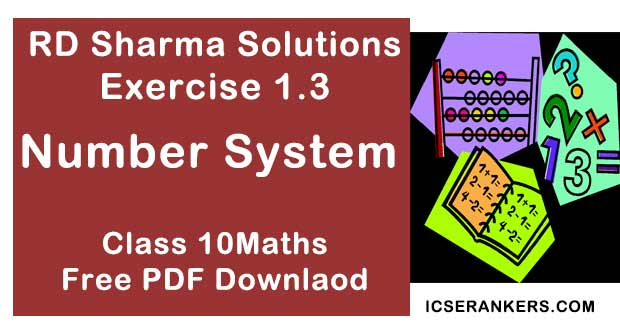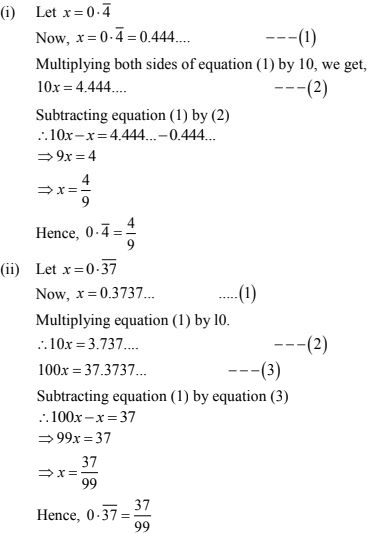# Chapter 1 Number System RD Sharma Solutions Exercise 1.3 Class 9 MathsChapter Name RD Sharma Chapter 1 Number System Exercise 1.3 Book Name RD Sharma Mathematics for Class 10 Other Exercises Exercise 1.1Exercise 1.2Exercise 1.4Exercise 1.5Exercise 1.6 Related Study NCERT Solutions for Class 10 Maths

### Exercise 1.3 Solutions

1. Express each of the following decimals in the form p/q .
(i) 0.39
(ii) 0.750
(iii) 2.15
(iv) 7.010
(v) 9.90
(vi) 1.0001

Solution

(i) We have,
0.39 = 39/100

(ii) We have,
0.750 = 750/1000 = (750 ÷ 250)/(1000 ÷ 250) = 3/4
∴ 0.750 = 3/4

(iii) We have
2.15 = 215/100 = (215 ÷ 5)/(100 ÷ 5) = 43/20
∴ 2.15 = 43/20

(iv) We have,
7.010 = 7010/1000 = (7010 ÷ 10)/(1000 ÷ 10) = 701/100
∴ 7010 = 701/100

(v) We have,
9.90 = 990/100 = (990 ÷ 10)/(100 ÷ 10) = 99/10
∴ 9.90 = 99/10

(vi) We have,
1.0001 = 10001/10000
∴ 1.0001 = 10001/10000

2. Express each of the following decimals in the form p/q.

(i) 0.4

(ii) 0.37

Solution